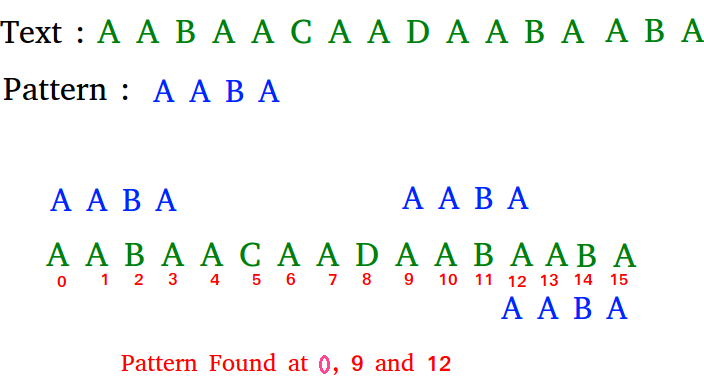# C# Program for KMP Algorithm for Pattern Searching

Given a text txt[0..n-1] and a pattern pat[0..m-1], write a function search(char pat[], char txt[]) that prints all occurrences of pat[] in txt[]. You may assume that n > m.

Examples:

```Input:  txt[] = "THIS IS A TEST TEXT"
pat[] = "TEST"
Output: Pattern found at index 10

pat[] =  "AABA"
Output: Pattern found at index 0
Pattern found at index 9
Pattern found at index 12```

## Recommended: Please solve it on “PRACTICE ” first, before moving on to the solution.

Pattern searching is an important problem in computer science. When we do search for a string in notepad/word file or browser or database, pattern searching algorithms are used to show the search results.

## C#

 `// C# program for implementation of KMP pattern ` `// searching algorithm ` `using` `System; ` ` `  `class` `GFG { ` ` `  `    ``void` `KMPSearch(``string` `pat, ``string` `txt) ` `    ``{ ` `        ``int` `M = pat.Length; ` `        ``int` `N = txt.Length; ` ` `  `        ``// create lps[] that will hold the longest ` `        ``// prefix suffix values for pattern ` `        ``int``[] lps = ``new` `int``[M]; ` `        ``int` `j = 0; ``// index for pat[] ` ` `  `        ``// Preprocess the pattern (calculate lps[] ` `        ``// array) ` `        ``computeLPSArray(pat, M, lps); ` ` `  `        ``int` `i = 0; ``// index for txt[] ` `        ``while` `(i < N) { ` `            ``if` `(pat[j] == txt[i]) { ` `                ``j++; ` `                ``i++; ` `            ``} ` `            ``if` `(j == M) { ` `                ``Console.Write(``"Found pattern "` `                              ``+ ``"at index "` `+ (i - j)); ` `                ``j = lps[j - 1]; ` `            ``} ` ` `  `            ``// mismatch after j matches ` `            ``else` `if` `(i < N && pat[j] != txt[i]) { ` `                ``// Do not match lps[0..lps[j-1]] characters, ` `                ``// they will match anyway ` `                ``if` `(j != 0) ` `                    ``j = lps[j - 1]; ` `                ``else` `                    ``i = i + 1; ` `            ``} ` `        ``} ` `    ``} ` ` `  `    ``void` `computeLPSArray(``string` `pat, ``int` `M, ``int``[] lps) ` `    ``{ ` `        ``// length of the previous longest prefix suffix ` `        ``int` `len = 0; ` `        ``int` `i = 1; ` `        ``lps = 0; ``// lps is always 0 ` ` `  `        ``// the loop calculates lps[i] for i = 1 to M-1 ` `        ``while` `(i < M) { ` `            ``if` `(pat[i] == pat[len]) { ` `                ``len++; ` `                ``lps[i] = len; ` `                ``i++; ` `            ``} ` `            ``else` `// (pat[i] != pat[len]) ` `            ``{ ` `                ``// This is tricky. Consider the example. ` `                ``// AAACAAAA and i = 7. The idea is similar ` `                ``// to search step. ` `                ``if` `(len != 0) { ` `                    ``len = lps[len - 1]; ` ` `  `                    ``// Also, note that we do not increment ` `                    ``// i here ` `                ``} ` `                ``else` `// if (len == 0) ` `                ``{ ` `                    ``lps[i] = len; ` `                    ``i++; ` `                ``} ` `            ``} ` `        ``} ` `    ``} ` ` `  `    ``// Driver program to test above function ` `    ``public` `static` `void` `Main() ` `    ``{ ` `        ``string` `txt = ``"ABABDABACDABABCABAB"``; ` `        ``string` `pat = ``"ABABCABAB"``; ` `        ``new` `GFG().KMPSearch(pat, txt); ` `    ``} ` `} ` ` `  `// This code has been contributed by Amit Khandelwal. `

Output:

```Found pattern at index 10
```

Please refer complete article on KMP Algorithm for Pattern Searching for more details!

My Personal Notes arrow_drop_up
Article Tags :

1

Please write to us at contribute@geeksforgeeks.org to report any issue with the above content.